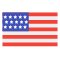enStyles
Categories
Trends
Old Interface
CollectionsView All
Drag icons
here

Divide
Collection
Divide
Collection
Divide
Collection
Divide
Collection
Divide
Collection
Divide
Collection
Divide
Collection
Divide
Collection
Divide
Collection
Divide
Collection
Multiplication
Collection
Multiplication
Collection
Multiplication
Collection
Multiplication
Collection
Multiplication
Collection
Multiplication
Collection
Multiplication
Collection
Multiplication
Collection
Multiplication
Collection
Multiplication
Collection
Multiplication
Collection
Multiplication
Collection
Less or Equal
Collection
Multiplication
Collection
Multiplication
Collection
Collection
Collection
Multiplication
Collection
Multiplication
Collection
Collection
Collection
Multiplication
Collection
Multiplication
Collection
Abacus
Collection
Abacus
Collection
Abacus
Collection
Abacus
Collection
Abacus
Collection
Collection
Abacus
Collection
Collection
Collection
Less or Equal
Collection
Collection
Abacus
Collection
Abacus
Collection
Abacus
Collection
Collection
Less or Equal
Collection
Collection
Less or Equal
Collection
Collection
Less or Equal
Collection
Abacus
Collection
Abacus
Collection
Multiply
Collection
Collection
Collection
Less or Equal
Collection
Less or Equal
Collection
Collection
Less or Equal
Collection
Collection
Less or Equal
Collection
Collection
Less or Equal
Collection
Collection
Less or Equal
Collection
Collection
Less or Equal
Collection
Collection
Less or Equal
Collection
Multiply
Collection
Multiply
Collection
Multiply
Collection
Multiply
Collection
Collection
Less or Equal
Collection
Collection
Less or Equal
Collection
Collection
Less or Equal
Collection
Collection
Less or Equal
Collection
Collection
Less or Equal
Collection
Collection
Less or Equal
Collection
Multiply
Collection
More or Equal
Collection
Multiply
Collection
Multiply
Collection
Multiply
Collection
Multiply
Collection
Multiply
Collection
Multiply
Collection
Multiply
Collection
Multiply
Collection
Multiply
Collection
Multiply
Collection
Multiply
Collection
Multiply
Collection
Multiply
Collection
Multiply
Collection
More or Equal
Collection
Multiply
Collection
Multiply
Collection
Multiply
Collection
Multiply
Collection
Multiply
Collection
Multiply
Collection
Multiply
Collection
More or Equal
Collection
More or Equal
Collection
More or Equal
Collection
More or Equal
Collection
More or Equal
Collection
More or Equal
Collection
More or Equal
Collection
More or Equal
Collection
Collections
0 set
Create new
Drag icons here